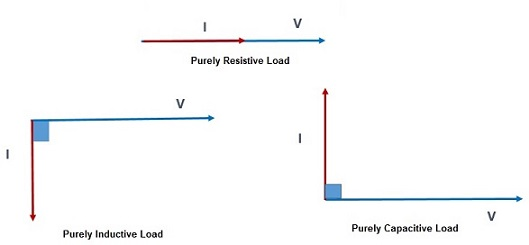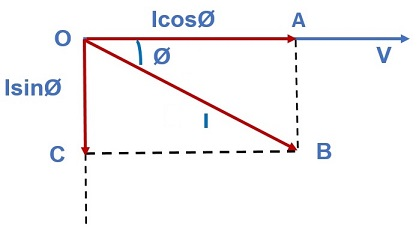# Active, Reactive and Apparent Power

### Active Power:

Active power is the real power consumed in an electrical circuit. It is the useful power which can be converted into other form of energy like heat energy in heater, light energy in bulb etc. It is also known as true or real power and measured in terms Watt, kW (Kilo Watt) or MW (1 Mega Watt = 106 Watt).

#### Significance:

It is required to perform different kind of useful work. Every appliance or loads require active power to run like Television, Motor, Refrigerator etc.

### Reactive Power:

Reactive power does not perform any real work. Here real work means that this power is cannot be utilized for heating, lighting or other useful purposes. It only pulsates back and forth in the circuit. It is measured in terms of kVAR (Kilo Volt Reactive) or MVAR (Mega Volt Reactive).

#### Significance:

Though reactive power does not perform any useful work, still it is necessary for the satisfactory operation electrical machine. It is required to build up magnetic field in the air gap of machine without which active power can neither be generated in generator nor consumed in motor.

### Apparent Power:

Apparent power is Volt Ampere of an electrical appliance or machine. If a machine is supplied with voltage V (rms) and current I (rms) is flowing through the machine, then it is the multiplication of rms voltage and current i.e. VI. It is measured in kVA or MVA.

Apparent Power, S = VI

#### Significance:

The losses in an electrical machine only depend on the voltage and the current. It does not depend on the power factor. Therefore apparent power gives an idea of losses in the machine.

### Derivation of Active and Reactive Power:

Electrical Load may either be Resistive, Inductive, Capacitive or their combinations. The natures of current flowing through these loads when connected to a voltage source are as follows:

• Purely resistive load takes current in phase with the applied voltage.
• Purely inductive load takes current lagging the applied voltage by 90 degree.
• Purely capacitive load takes current leading the applied voltage by 90 degree.Thus the angle between voltage and current for purely resistive, inductive and capacitive loads are 0º, 90º and 90º degree respectively. But when a load comprises of inductance and resistance, the current I through the load will be lagging w.r.t voltage V by some angle Ø as shown below.This current I can now be resolved into two components:

• Along the voltage i.e. IcosØ
• Perpendicular to the voltage i.e. IsinØ

#### Active Current:

The component of load current along the voltage is called active current. A load consumes active power due to this component of current. Hence, the true or real power is given as

Real Power = Voltage x (Active Current)

= VIcosØ

#### Reactive Current:

The component of load current perpendicular to the voltage is called reactive current. Reactive power in a circuit is due to this component of current. Hence,

Reactive Power, Q = Voltage x (Reactive Current)

= VIsinØ

 Active / Real Power Reactive Power Apparent Power VIcosØ VIsinØ VI

Why Resistance only consume Real Power?

As discussed earlier in this post the angle Ø for pure resistance is 0º and that for inductor and capacitor is 90º. This means that a pure resistance will only consume active power as VIcos0 = VI and no reactive power since VIsin0 = 0.

Why Inductor and Capacitor do not consume any Real Power?

A pure inductor & capacitor only consumes reactive power as VIsin90 = VI and no active power as VIcos90 = 0. This can also be understood in a different way. Whatever power is received from the source in one half cycle by these circuit elements, the same amount of power is being returned to the source in next half cycle. Therefore, the average power consumption for a complete cycle is zero. Hence no true power is consumed.

This site uses Akismet to reduce spam. Learn how your comment data is processed.